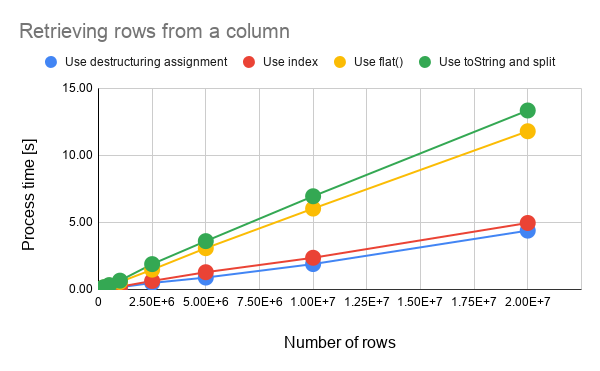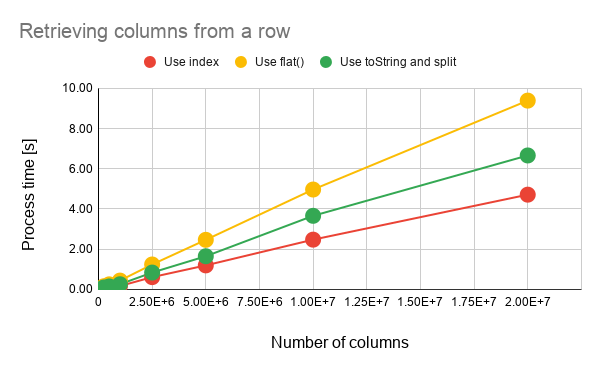# Benchmark: Process Costs for Retrieving Values from Arrays for Spreadsheet using Google Apps Script

Gists

## Introduction

Here, I would like to report the process costs for retrieving the values from the arrays for Spreadsheet using Google Apps Script (GAS). When Spreadsheet is used with Google Apps Script, we have the following situations.

1. Retrieve values from the multiple rows in a column.

2. Retrieve values from the multiple columns in a row.

When the values are retrieved from above situations, it is required to retrieve the values from 1 dimensional array in the 2 dimensional array. In this report, the process costs for retrieving the values from the 2 dimensional array of above situations have been measured.

As the result, it was found that when the values are retrieved from the arrays with n rows in a column and n columns in a row, to use the destructuring assignment and to use the index are suitable, respectively.

## Experimental procedure

In this report, the following sample arrays were used for measuring the process costs for retrieving the values from the arrays with the multiple rows in a column and the multiple columns in a row.

• The multiple rows in a column: [["a"],["a"],["a"],,,]

• The number of rows were changed from 0 to 20,000,000.
• The multiple columns in a row: [["a","a","a",,,]]

• The number of columns were changed from 0 to 20,000,000.

The values of all elements are the same with the string value of "a". The script for creating these sample arrays can be seen at Appendix. As the simple test for this situation, the string value of "b" is added to each element. You can see the sample scripts for measuring the cost as follows. Also, you can see them at Appendix.

• Scripts for n rows in a column

• Using destructuring assignment

const pattern1 = (ar) => ar.map(([e]) => e + "b");

• Using index

const pattern2 = (ar) => ar.map((e) => e + "b");

• Using toString() and split(): This is for both n rows in a column and n columns in a row.

const pattern3 = (ar) =>
ar
.toString()
.split(",")
.map((e) => e + "b");

• Using flat(): This is for both n rows in a column and n columns in a row.

const pattern4 = (ar) => ar.flat().map((e) => e + "b");

• Scripts for n columns in a row

• Using index

const pattern1 = (ar) => ar.map((e) => e + "b");

• Using toString() and split(): This is for both n rows in a column and n columns in a row.

const pattern2 = (ar) =>
ar
.toString()
.split(",")
.map((e) => e + "b");

• Using flat(): This is for both n rows in a column and n columns in a row.

const pattern3 = (ar) => ar.flat().map((e) => e + "b");


The output value from the each script is ["ab","ab","ab",,,].

In this experiment, the sample scripts for GAS were used with enabling V8 runtime.

By the way, at GAS, the processing time is not stable as you know. So the average value for more than 200 times measurements was used for each data point which is shown by figures. At this time, the fluctuation of the average values was less than 1 %. I worry that each detailed-data point at my environment might be different from that at other user’s environment. But I think that the trend of this result can be used.

## Results and discussionsFig. 1. Process time dependence on number of rows. The number of columns is 1.

From Fig. 1, it is found the following results.

1. In the case for retrieving the values from the array with n rows in a column, the process cost is linearly increased with increasing the number of rows.

2. The method with the lowest cost is to use the destructuring assignment.

• The cost using the index is a bit higher than that using the destructuring assignment
3. The highest cost is to use toString and split.

• The cost using toString and split is a bit higher than that using flat.
4. When the destructuring assignment is used for retrieving the values from the array with n rows in a column, the cost can be reduced with about 76 %, 71 % and 25 % from the method for using toString and split, flat and the index, respectively.Fig. 2. Process time dependence on number of column. The number of rows is 1.

From Fig. 2, it is found the following results.

1. In the case for retrieving the values from the array with n columns in a row, the process cost is linearly increased with increasing the number of rows.

2. The method with the lowest cost is to use the index.

3. The highest cost is to use flat.

4. When the index is used for retrieving the values from the array with n columns, the cost can be reduced with about 52 % and 30 % from the method for using flat, toString and split, respectively.

From Figs. 1 and 2, it is found the following results.

1. The method for using the index can be used for both situations.

2. In the case of method for using the index, the process costs are almost the same for both n rows in a column and n columns in a row, when the total number of elements in the array.

3. In the case of method for using flat and using toString and split, the process cost for processing n columns in a row is lower than that for processing n rows in a column, when the total number of elements in the array.

• The difference of the cost for processing between n rows in a column and n columns in a row is larger for the method using toString and split.

## Summary

In this report, the process cost for retrieving the values from the 2 dimensional array of above situations have been measured. I could obtain the following results.

1. When the values are retrieved from an array with n rows in a column, to use the destructuring assignment is suitable.

2. When the values are retrieved from an array with n columns in a row, to use the index is suitable.

3. The cost of method for using toString and split strongly depends on the array structure. The cost for processing n columns in a row is much lower than that for processing n rows in a column.

As a note, I have to describe that this is the result for Google Apps Script. For other languages, this result might be difference. And also, the process cost of this report might be modified by future update of Google.

## Appendix

### Scripts for creating sample arrays

Scripts for creating the sample array are as follows.

// n rows in a column: Create an array of [["a"],["a"],["a"],,,]
const sampleArray1 = (row) => Array(row).fill(["a"]);

// n columns in a row: Create an array of [["a","a","a",,,]]
const sampleArray2 = (row) => [Array(row).fill("a")];


### Scripts for measuring process cost for n rows in a column

Scripts for measuring the process cost for n rows in a column are as follows.

// Using destructuring assignment
const pattern1 = (ar) => ar.map(([e]) => e + "b");

// Using index
const pattern2 = (ar) => ar.map((e) => e + "b");

// Using toString and split: This is for both n rows in a column and n columns in a row.
const pattern3 = (ar) =>
ar
.toString()
.split(",")
.map((e) => e + "b");

// Using flat: This is for both n rows in a column and n columns in a row.
const pattern4 = (ar) => ar.flat().map((e) => e + "b");


### Scripts for measuring process cost for n columns in a row

Scripts for measuring the process cost for n columns in a row are as follows.

// Using index
const pattern1 = (ar) => ar.map((e) => e + "b");

// Using toString and split: This is for both n rows in a column and n columns in a row.
const pattern2 = (ar) =>
ar
.toString()
.split(",")
.map((e) => e + "b");

// Using flat: This is for both n rows in a column and n columns in a row.
const pattern3 = (ar) => ar.flat().map((e) => e + "b");